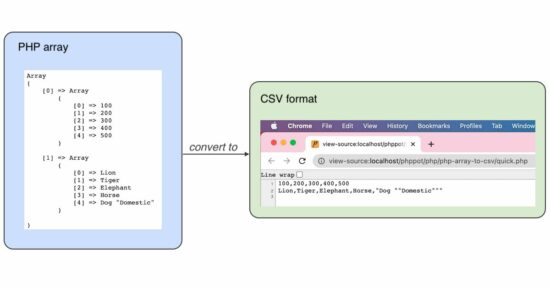# Convert PHP Array to CSV

This tutorial is to convert a PHP array to a CSV file or format. It has two examples of implementing this conversion.

1. A quick example is that parses a PHP array dataset to a CSV and prints the CSV data on the browser.
2. Another example is to convert the database array to CSV and download the .csv file.

## Example 1: Quick example

The below code uses the PHP fputcsv() function to convert the array to CSV. This function requires the following arguments to specify.

1. A target CSV file.
2. Iterating array pinter to put the CSV row.
3. Data delimiter.
4. Data enclosure.
``````<?php
\$array = array(
array(100, 200, 300, 400, 500),
array('Lion', 'Tiger', 'Elephant', 'Horse', 'Dog "Domestic"'),
);
\$csvDelimiter = ',';
\$csvEnclosure = '"';

// to physically write to a file in disk
\$csvFile = fopen('example.csv', 'w+');

// to write to temp
\$csvFile = fopen('php://temp', 'r+');

foreach (\$array as \$line) {
fputcsv(\$csvFile, \$line, \$csvDelimiter, \$csvEnclosure);
}
rewind(\$csvFile);

// to display CSV file contents on browser
\$contents = '';
while (!feof(\$csvFile)) {
}
fclose(\$csvFile);
echo \$contents;
?>
``````This quick example creates an input array for converting into CSV. This is a multidimensional array that has the dataset.

This code sets the delimiter and enclosure before converting the input array.

It iterates the array and calls fputcsv() on each iteration to form the data row.

Get the code from the linked article if you want to convert CSV to a PHP array.

This PHP code is to learn how to convert an array of data from the database to CSV. Previously, we have seen how to handle CSV in PHP to read, write, import, and export operations with a database.

In this example, code connects the database and reads the results into an array. Then, it creates a PHP file output stream to put the CSV data row by row.

First, the code sets the array of column headers. Then, it iterates the database result array and calls fputcsv() on each row of data.

``````<?php
// PHP code will connect to a database
// Read from a table and get result
// Formulate the result as an array to use in a convenient form
// Iterate the array
// Use PHP's function fputcsv and write the array elements
// to a CSV file
\$mysqli = new mysqli("localhost", "root", "", "db_phppot_examples");
\$i = 0;
\$query = "SELECT id, name, type FROM tbl_php_array_to_csv";
if (\$resultArray = \$mysqli->query(\$query)) {
while (\$record = \$resultArray->fetch_array()) {
\$animalArray[\$i]['id'] = \$record['id'];
\$animalArray[\$i]['name'] = \$record['name'];
\$animalArray[\$i]['type'] = \$record['type'];
\$i++;
}
}
\$fileOut = fopen("php://output", 'w') or die("Unable open php://output");

// writing the first CSV record as the column labels
// Refer: https://www.php.net/manual/en/function.fputcsv.php
fputcsv(\$fileOut, array('id', 'name', 'type'));

// writing array elements as CSV file records one by one
foreach (\$animalArray as \$animal) {
fputcsv(\$fileOut, \$animal);
}
fclose(\$fileOut) or die("Unable to close php://output");
?>
``````

### Database script

This database script imports the table structure and data to run this example.

You may also try this code with a different database table. It requires only minor code changes to edit the new table’s column names.

``````--
-- Database: `db_phppot_examples`
--

-- --------------------------------------------------------

--
-- Table structure for table `tbl_php_array_to_csv`
--

CREATE TABLE `tbl_php_array_to_csv` (
`id` int NOT NULL,
`name` varchar(255) NOT NULL,
`type` varchar(255) NOT NULL
);

--
-- Dumping data for table `tbl_php_array_to_csv`
--

INSERT INTO `tbl_php_array_to_csv` (`id`, `name`, `type`) VALUES
(1, 'Lion', 'Wild'),
(3, 'Dog', 'Domestic'),
(4, 'Tiger', 'Wild');

--
-- Indexes for dumped tables
--

--
-- Indexes for table `tbl_php_array_to_csv`
--
ALTER TABLE `tbl_php_array_to_csv`

--
-- AUTO_INCREMENT for dumped tables
--

--
-- AUTO_INCREMENT for table `tbl_php_array_to_csv`
--
ALTER TABLE `tbl_php_array_to_csv`
MODIFY `id` int NOT NULL AUTO_INCREMENT, AUTO_INCREMENT=6;
``````

## Uses of PHP array to CSV conversion

### (1) Database table export

We can use this code export database to a CSV file. It is for taking a backup of a database table.

If you want to create a PHP program to take a complete database backup into an excel, the linked article has the code.

### (2) Format data into a comma-separated value

It helps to convert various data sources and create a dumb in a unified CSV format.

In case of loading more data from an array into an existing CSV, this code will be helpful.